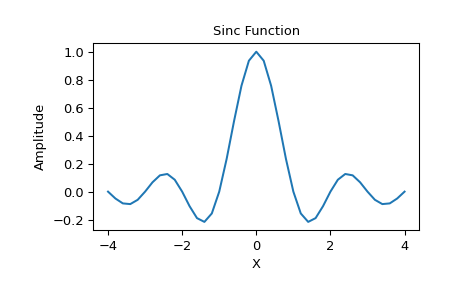# scipy.special.sinc¶

scipy.special.sinc(x)

Return the normalized sinc function.

The sinc function is $$\sin(\pi x)/(\pi x)$$.

Note

Note the normalization factor of pi used in the definition. This is the most commonly used definition in signal processing. Use sinc(x / np.pi) to obtain the unnormalized sinc function $$\sin(x)/(x)$$ that is more common in mathematics.

Parameters
xndarray

Array (possibly multi-dimensional) of values for which to to calculate sinc(x).

Returns
outndarray

sinc(x), which has the same shape as the input.

Notes

sinc(0) is the limit value 1.

The name sinc is short for “sine cardinal” or “sinus cardinalis”.

The sinc function is used in various signal processing applications, including in anti-aliasing, in the construction of a Lanczos resampling filter, and in interpolation.

For bandlimited interpolation of discrete-time signals, the ideal interpolation kernel is proportional to the sinc function.

References

1

Weisstein, Eric W. “Sinc Function.” From MathWorld–A Wolfram Web Resource. http://mathworld.wolfram.com/SincFunction.html

2

Wikipedia, “Sinc function”, https://en.wikipedia.org/wiki/Sinc_function

Examples

>>> import matplotlib.pyplot as plt
>>> x = np.linspace(-4, 4, 41)
>>> np.sinc(x)
array([-3.89804309e-17,  -4.92362781e-02,  -8.40918587e-02, # may vary
-8.90384387e-02,  -5.84680802e-02,   3.89804309e-17,
6.68206631e-02,   1.16434881e-01,   1.26137788e-01,
8.50444803e-02,  -3.89804309e-17,  -1.03943254e-01,
-1.89206682e-01,  -2.16236208e-01,  -1.55914881e-01,
3.89804309e-17,   2.33872321e-01,   5.04551152e-01,
7.56826729e-01,   9.35489284e-01,   1.00000000e+00,
9.35489284e-01,   7.56826729e-01,   5.04551152e-01,
2.33872321e-01,   3.89804309e-17,  -1.55914881e-01,
-2.16236208e-01,  -1.89206682e-01,  -1.03943254e-01,
-3.89804309e-17,   8.50444803e-02,   1.26137788e-01,
1.16434881e-01,   6.68206631e-02,   3.89804309e-17,
-5.84680802e-02,  -8.90384387e-02,  -8.40918587e-02,
-4.92362781e-02,  -3.89804309e-17])

>>> plt.plot(x, np.sinc(x))
[<matplotlib.lines.Line2D object at 0x...>]
>>> plt.title("Sinc Function")
Text(0.5, 1.0, 'Sinc Function')
>>> plt.ylabel("Amplitude")
Text(0, 0.5, 'Amplitude')
>>> plt.xlabel("X")
Text(0.5, 0, 'X')
>>> plt.show()#### Previous topic

scipy.special.exprel

#### Next topic

Statistical functions (scipy.stats)